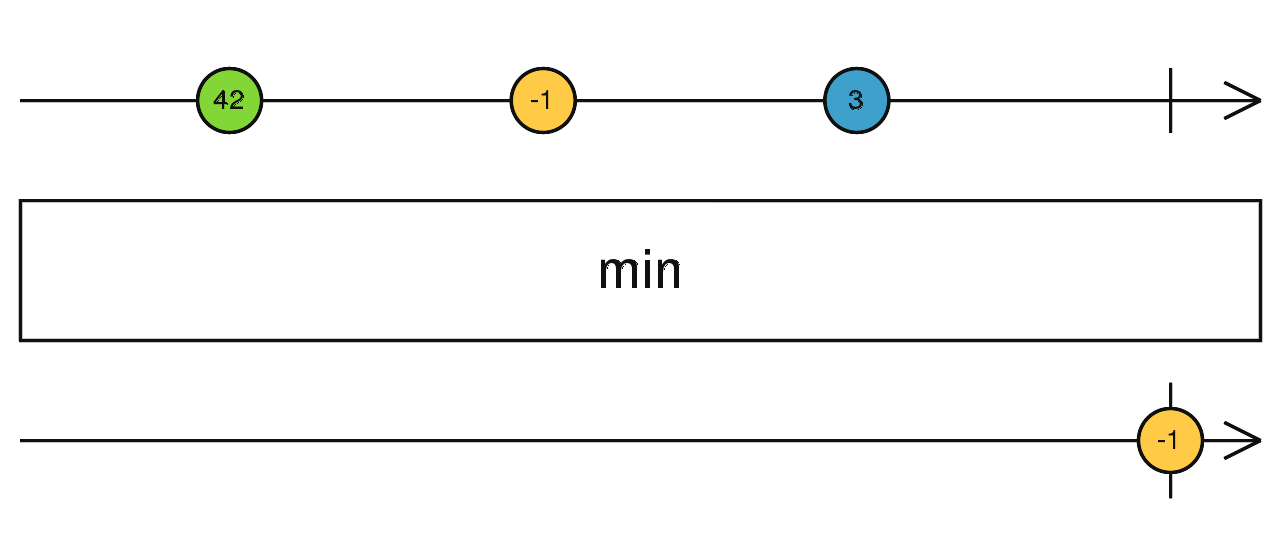/RxJS

# min

`function` `stable`

The Min operator operates on an Observable that emits numbers (or items that can be compared with a provided function), and when source Observable completes it emits a single item: the item with the smallest value.

### `min<T>(comparer?: (x: T, y: T) => number): MonoTypeOperatorFunction<T>`

#### Parameters

 comparer Optional. Default is `undefined`. Optional comparer function that it will use instead of its default to compare the value of two items.

#### Returns

`MonoTypeOperatorFunction<T>`: An Observable that emits item with the smallest value.

## Description## Examples

Get the minimal value of a series of numbers

```import { of } from 'rxjs';
import { min } from 'rxjs/operators';

of(5, 4, 7, 2, 8).pipe(
min(),
)
.subscribe(x => console.log(x)); // -> 2```

Use a comparer function to get the minimal item

```import { of } from 'rxjs';
import { min } from 'rxjs/operators';

interface Person {
age: number,
name: string
}
of<Person>(
{age: 7, name: 'Foo'},
{age: 5, name: 'Bar'},
{age: 9, name: 'Beer'},
).pipe(
min<Person>( (a: Person, b: Person) => a.age < b.age ? -1 : 1),
)
.subscribe((x: Person) => console.log(x.name)); // -> 'Bar'```